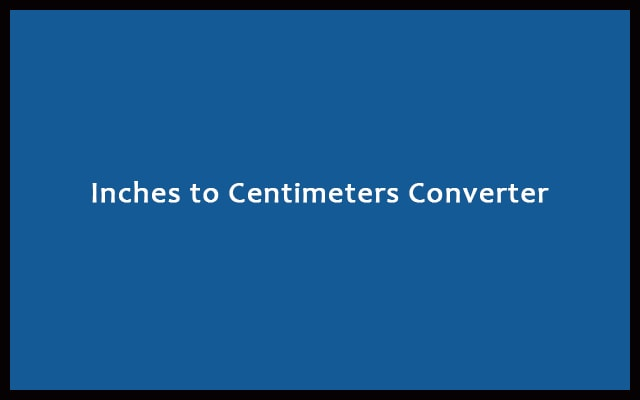# Inches to Cm – Inches to Centimeters Converter Calculator

Inch and Centimeter are two length measuring units. Both have their individual importance. Sometimes you need to convert Inches to Cm using our tool.

Formula: 1 inch = 2.54 cm

Centimeters Output:
Meters + Centimeters Output:
m cm
Full Calculation:
RelatedMeasuring in daily life is done using instruments. Like scale which comes to different units. Most of us are using the centimeter scale. We use it to draw lines and figures of a specific length. Measurement is done when you need to find the distance between two points. It depends upon your requirements. Which unit inch or centimeter you prefer. Using our inches to cm converter you can change inches to centimeters easily. So, you can use it anywhere and anytime.

## Inches to Cm Conversion Calculator

For example:

• For buying a pair of shoes, we need to measure the size of our feet.
• Before traveling we sometimes find out the distance between our point to the destination point. For distance calculation. Therefore, bigger units like kilometer and miles are used.
• While purchasing clothes, measurement is required. For the perfect fitting, we need an exact measurement. So, measuring tapes and scales are used. They may be in inches as well as in centimeter.
• Lastly, in construction works, perfect measurement plays an important role. Therefore, people use very accurate instruments like laser distance measuring meter. Also, they have nearly zero error.

### Inch vs Centimeter Measurement System

Both inch and centimeters are using for distance measuring. Like measuring length, width, height, etc. A centimeter is 1/100th part of 1 meter. Similarly, 1 meter is equal to 100 centimeters.

A centimeter is an SI unit. However, it is only suitable for measuring small distances. For larger distances, we use the meter. For a very large distance, we use a kilometer. It is not that you can’t use centimeter for large distance measurement. It is not preferred because the value becomes very large. So, it becomes difficult to present and store. A centimeter symbol is “cm”.

Inch is 1/12th part of afoot. It is mainly using to represent screen sizes. Inch is symbolized by “in”. Also, It is a popular unit of measurement. In ancient times barleycorn was used. One inch consisted of three dry barleycorn connected end to end. Nowadays there are instruments for inch measurement. It is more accurate and easier. Inch is not considered in SI units. Then it also plays a very important role in real life.

#### Formula/Relationship Between Inch and Cm

1 inch = 2.54 centimeters

Thus,

• 2 inch will be 2 x 2.54 = 5.08 centimeters.
• 3 inch will be 3 x 2.54 = 7.62 centimeter.

This is the way inches to cm conversion takes place.

## How to convert inches to cm?

As we discussed earlier. 1 inch is equal to 2.54 cm. Hence, using this concept we will calculate for inches. For any inch convert into centimeter, we have to multiply it by 2.54.

Let’s make this clear by seeing some examples.

Example 1:

Convert 3.52 inches to cm.
Here we multiply 3.52 with 2.54 to get 8.9408 centimeters. Therefore, 3.52 inches = 8.9408 cm.

Example 2:

Convert 0.562 inches to cm.
Here we multiply 0.562 with 2.54 to get 1.42748 centimeter. Therefore, 0.562 inches = 1.42748 cm.

## Uses of Inches to Centimeter Converter

There are some situations where we need to fill data whether with inch or cm. If it requires cm and you have recorded in inch. Then our converter will be very helpful at such time. As it can convert inches to centimeters.

Various mathematical and scientific calculations require that all units must be the same. Hence, by using a converter. You can make all the units the same. For ease calculation and accurate results.

#### Advantages of Inches to Centimeter Converter

1. For fast conversion. It is preferable to go for a converter. It completes the process in less than a second. Just fill the inch and press convert. It saves time and effort. In manual calculation, you have to spend a lot of time.
2. For accurate conversion. It is preferable to go for a converter. It gives a 100% correct results. As it runs on predefined algorithms. Humans can do mistakes. Computers can never.

#### How to Use the Converter?

First of all, connect to the Internet. Because it is an online tool. Then open your web browser. Go to the Inches to Centimeter Converter. It is the tool that will convert inches to the centimeter. There you will find an input box to enter inches. Type the inch value there. Press “Convert” button to convert inches to cm. As a result, The centimeter result will be displayed below. Lastly, For new conversion just press the “Reset” button.

#### Inches to Centimeters Conversion Table

Inches (“) Centimeters (cm)
0.01 inch 0.0254 cm
1/64 inch 0.0396875 cm
1/32 inch 0.079375 cm
1/16 inch 0.15875 cm
0.1 inch 0.254 cm
1/8 inch 0.3175 cm
1/4 inch 0.635 cm
1/2 inch 1.27 cm
1 inch 2.54 cm
2 inch 5.08 cm
3 inch 7.62 cm
4 inch 10.16 cm
5 inch 12.70 cm
6 inch 15.24 cm
7 inch 17.78 cm
8 inch 20.32 cm
9 inch 22.86 cm
10 inch 25.40 cm
20 inch 50.80 cm
30 inch 76.20 cm
40 inch 101.60 cm
50 inch 127.00 cm
60 inch 152.40 cm
70 inch 177.80 cm
80 inch 203.20 cm
90 inch 228.60 cm
100 inch 254.00 cm